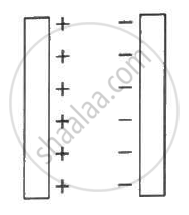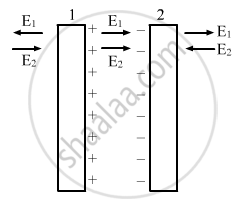Department of Pre-University Education, KarnatakaPUC Karnataka Science Class 12
Share

# Two Large Conducting Plates Are Placed Parallel to Each Other and They Carry Equal and Opposite Charges with Surface Density σ as Shown in the Figure. - Physics

ConceptElectric Charges

#### Question

Two large conducting plates are placed parallel to each other and they carry equal and opposite charges with surface density σ as shown in the figure. Find the electric field (a) at the left of the plates (b) in between the plates and (c) at the right of the plates.#### Solution

Given:-

Surface charge density on the plates = σ

The electric field due to plate 1, E1 = sigma/(2∈_0)

The electric field due to plate 2, E2 = sigma/(2∈_0)

(a) The strength of the electric field due to both the plates will be same but their directions will be opposite to each other on any point at the left of the two plates.

Thus, the net electric field at a point on the left of plate1 = sigma /(2∈_0) - sigma/(2∈_0) = 0

(b) Here the direction of the fields will be same. So, they will add up to give the resultant field in this region.

Total electric field:-

sigma/(2∈_0) + sigma /(2∈_0) = sigma/∈_0

(c) The strength of the electric field due to both the plates will be same but their directions will be opposite to each other at any point on the right of the two plates.

Thus, the net electric field at a point on the left of plate 2 = sigma /(2∈_0) -  sigma/(2∈_0) = 0Is there an error in this question or solution?

#### Video TutorialsVIEW ALL 

Solution Two Large Conducting Plates Are Placed Parallel to Each Other and They Carry Equal and Opposite Charges with Surface Density σ as Shown in the Figure. Concept: Electric Charges.
S